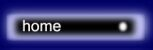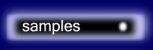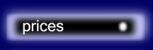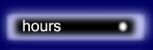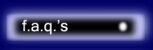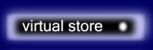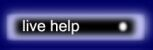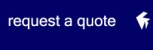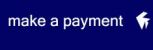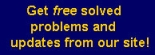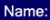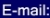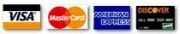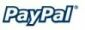# The place to find MATH HOMEWORK HELP online

For a list of free math problems, click on the link (see the snapshot below)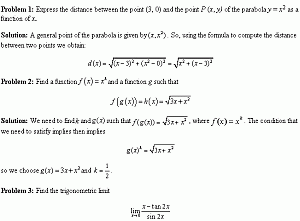Here we provide you with list of solved math problems, for you to use.

Problem 1

Problem 2

Problem 3

Problem 4

Problem 5

Problem 6

Problem 7

Problem 8

Problem 9

Problem 10

Problem 11

Problem 12

Problem 13

Problem 14

Problem 15

Problem 16

Problem 17

Problem 18

Problem 19

Problem 20

Problem 21

Problem 22

Problem 23

Problem 24

Problem 25

Problem 26

Problem 27

 The ways you can submit your problems to us for a FREE quote are: By e-mail: You send us an e-mail with your questions. It can be any type of file, doc, pdf, xls, or a scanned image of the problems. The turnaround is 24-48 hours. By fax: We'll give you our fax number on request.

MGT - Your source of Math and Statistics Homework Help

 Statistics Help   |   Calculus Homework Online   |   Operations Management Help   |   Request a Quote     |     Calculus II and III     |    Linear Algebra     |    Statistical Analysis - Statistics Solutions Contact us at mgtutor@gmail.com © 2005-2017 MyGeekyTutor.com - Your preferred help with math homework All rights reserved